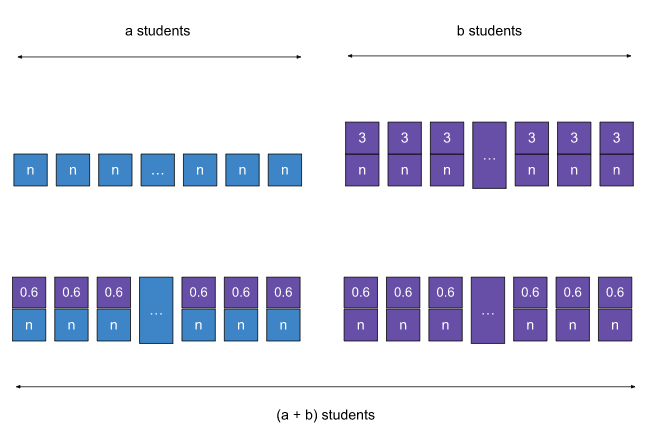# CAT 2022 Question Paper | Quant Slot 1

###### CAT Previous Year Paper | CAT Quant Questions | Question 20

CAT 2022 Quant was dominated by Arithmetic followed by Algebra. In Arithmetic, the questions were dominated by topics like Speed-time-distance, Mixture and Alligations. This year, there was a surprise. The questions from Geometry were relatively on the lower side as compared to the previous years. There were 8 TITA Qs this year. Overall this section was at a medium level of difficulty.

Question 20 : The average weight of students in a class increases by 600 gm when some new students join the class. If the average weight of the new students is 3 kg more than the average weight of the original students, then the ratio of the number of original students to the number of new students is

1. 1 : 2
2. 3 : 1
3. 1 : 4
4. 4 : 1

## Best CAT Coaching in Chennai

#### CAT Coaching in Chennai - CAT 2022Limited Seats Available - Register Now!

Let there be ‘a’ number of students originally in the class and their average weight be ‘n’ kgs.
Let ‘b’ number of students join the class having an average weight of ‘n + 3’ kgs.
This excess weight of 3 kgs brought in by each new student is shared among all the students such that each of them increases by 0.6 kgs.3b = 0.6(a + b)
3b = 0.6 a + 0.6 b
2.4b = 0.6a
a = 4b
a : b = 4 : 1
The ratio of the number of original students to the number of new students is 4 : 1

##### The answer is '4 : 1'

Choice D is the correct answer.

###### CAT Coaching in ChennaiCAT 2023

Classroom Batches Starting Now! @Gopalapuram

###### Best CAT Coaching in Chennai Introductory offer of 5000/-

Attend a Demo Class

##### Where is 2IIM located?

2IIM Online CAT Coaching
A Fermat Education Initiative,
58/16, Indira Gandhi Street,
Kaveri Rangan Nagar, Saligramam, Chennai 600 093

##### How to reach 2IIM?

Mobile: (91) 99626 48484 / 94459 38484
WhatsApp: WhatsApp Now
Email: info@2iim.com